# 使用Python进行异常检测

#### 公式和过程

1. 使用以下公式计算平均值：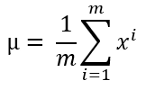1. 使用以下公式计算方差：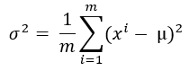1. 现在，用这个概率公式计算每个训练例子的概率。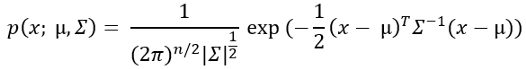1. 我们现在需要找到概率的临界值。正如我前面提到的，如果一个训练例子的概率很低，那就是一个异常的例子。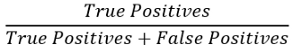False Positives（假正例）当算法检测到一个异常的例子，但在实际情况中，它不是异常的，就会出现误报。

False Negative（假反例）是指算法检测到的一个例子不是异常的，但实际上它是一个异常的例子。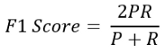#### 异常检测算法

https://github.com/rashida048/Machine-Learning-With-Python/blob/master/ex8data1.xlsx

import pandas as pd
import numpy as np

df = pd.read_excel('ex8data1.xlsx', sheet_name='X', header=None)
df.head()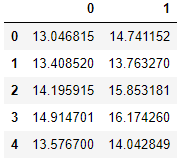plt.figure()
plt.scatter(df, df)
plt.show()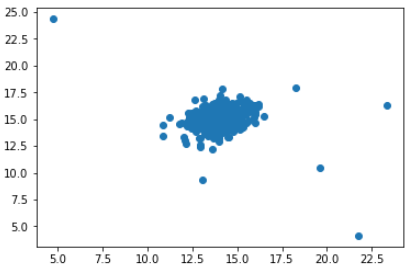m = len(df)

s = np.sum(df, axis=0)
mu = s/m
mu

0    14.112226
1    14.997711
dtype: float64

vr = np.sum((df - mu)**2, axis=0)
variance = vr/m
variance

0    1.832631
1    1.709745
dtype: float64

var_dia = np.diag(variance)
var_dia

array([[1.83263141, 0.        ],
[0.        , 1.70974533]])

k = len(mu)
X = df - mu
p = 1/((2*np.pi)**(k/2)*(np.linalg.det(var_dia)**0.5))* np.exp(-0.5* np.sum(X @ np.linalg.pinv(var_dia) * X,axis=1))
p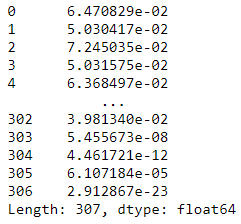cvx = pd.read_excel('ex8data1.xlsx', sheet_name='Xval', header=None)
cvx.head()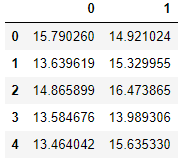cvy = pd.read_excel('ex8data1.xlsx', sheet_name='y', header=None)
cvy.head()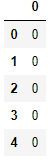y = np.array(cvy)

# 数组的一部分
array([,
,
,
,
,
,
,
,
,

p.describe()

count    3.070000e+02
mean     5.905331e-02
std      2.324461e-02
min      1.181209e-23
25%      4.361075e-02
50%      6.510144e-02
75%      7.849532e-02
max      8.986095e-02
dtype: float64

def tpfpfn(ep):
tp, fp, fn = 0, 0, 0
for i in range(len(y)):
if p[i] <= ep and y[i] == 1:
tp += 1
elif p[i] <= ep and y[i] == 0:
fp += 1
elif p[i] > ep and y[i] == 1:
fn += 1
return tp, fp, fn

eps = [i for i in p if i <= p.mean()]

len(eps)

133

def f1(ep):
tp, fp, fn = tpfpfn(ep)
prec = tp/(tp + fp)
rec = tp/(tp + fn)
f1 = 2*prec*rec/(prec + rec)
return f1

f = []
for i in eps:
f.append(f1(i))
f

[0.14285714285714285,
0.14035087719298248,
0.1927710843373494,
0.1568627450980392,
0.208955223880597,
0.41379310344827586,
0.15517241379310345,
0.28571428571428575,
0.19444444444444445,
0.5217391304347826,
0.19718309859154928,
0.19753086419753085,
0.29268292682926833,
0.14545454545454545,

f分数通常在0到1之间，其中f1得分越高越好。所以，我们需要从刚才计算的f分数列表中取f的最高分数。

np.array(f).argmax()

131

e = eps
e

6.107184445968581e-05

#### 找出异常实例

label = []
for i in range(len(df)):
if p[i] <= e:
label.append(1)
else:
label.append(0)
label

[0,
0,
0,
0,
0,
0,
0,
0,
0,
0,

df['label'] = np.array(label)
df.head()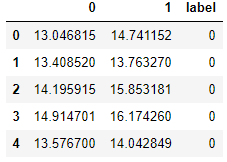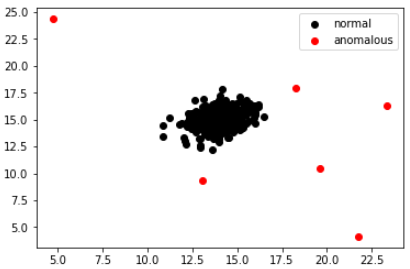#### 结论

http://panchuang.net/

sklearn机器学习中文官方文档：
http://sklearn123.com/

http://docs.panchuang.net/